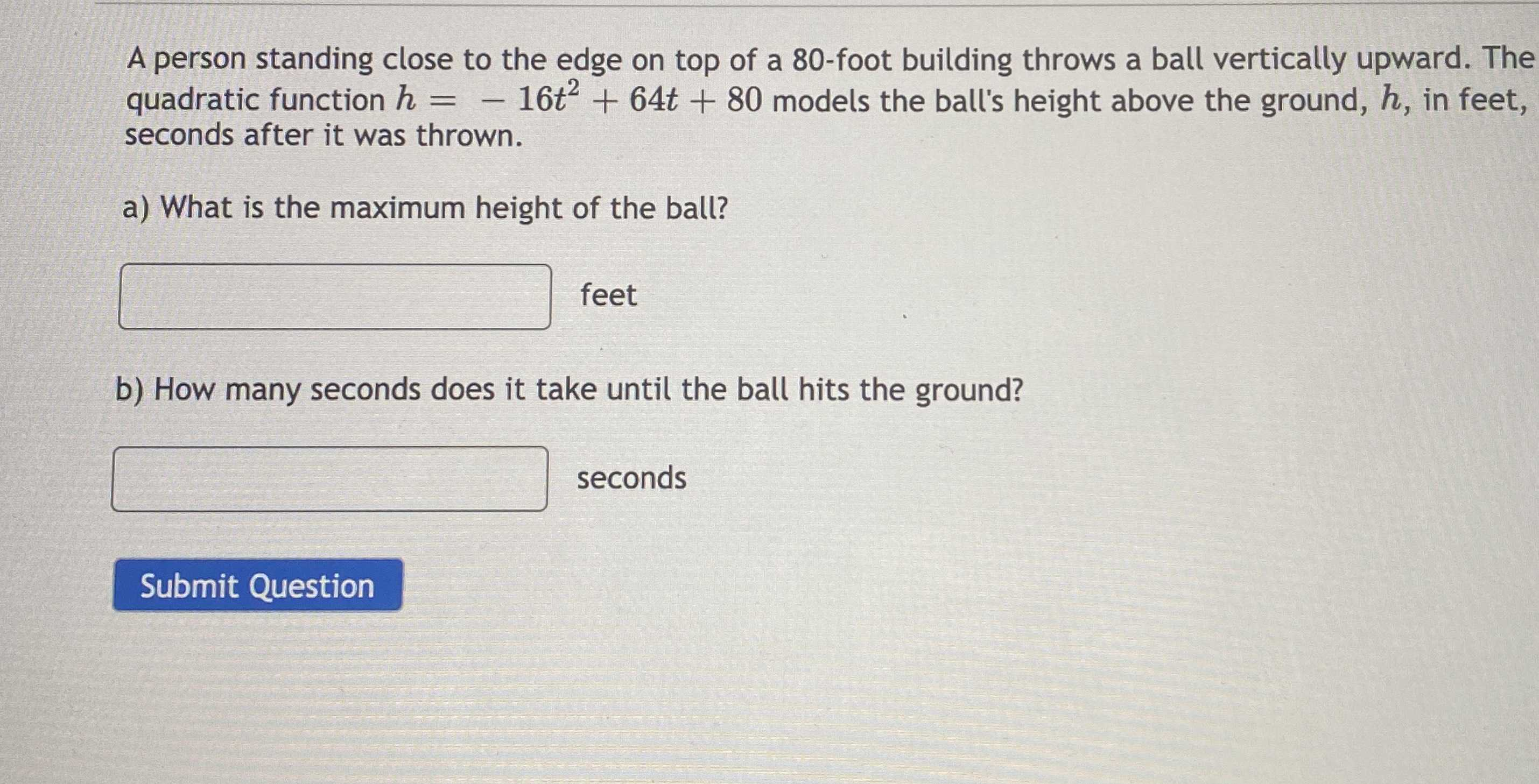### Still have math questions?

Algebra
QuestionA person standing close to the edge on top of a $$80$$ -foot building throws a ball vertically upward. The quadratic function $$h = - 16 t ^ { 2 } + 64 t + 80$$ models the ball's height above the ground, $$h$$ , in feet, seconds after it was thrown.

a) What is the maximum height of the ball?

__________feet

b) How many seconds does it take until the ball hits the ground?

___________seconds